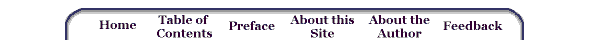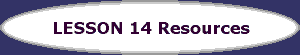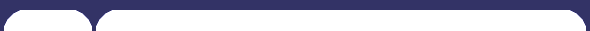xAlternate Versions of Figures
 Here is the version of Figure 14.9 that uses the onchange() event handler rather than a button to call the function. Here is the version of Figure 14.12 that uses the onchange() event handler rather than a button to call the function.

Source Files for Figures
 Figure 14.1 Figure 14.4 Figure 14.5 Figure 14.7 Figure 14.9 Figure 14.12 Section 14.7 Figure 14.14

Figures as They Appear in the Text

Support for
Homework Problems
 Exercise 1 Exercise 2 Exercise 5 Exercise 8 Exercise 9 Exercise 10 Exercise 12

 Extra Exercises